# Michael E Hendersongen_feedback_link(leftr, rightr);

## contact information

Principal Research Staff Member, Applied Mathematics
Thomas J. Watson Research Center, Yorktown Heights, NY USA

### Professional Associations

Professional Associations:  ACM  |  IEEE   |  Society for Industrial and Applied Mathematics  |  Tau Beta Pi - The Engineering Honor Society

### Multifario example -- the coaxially clamped twisted rod

This is joint work with Sebastien Neukirch

Classification of the spatial equilibria of the clamped elastica: numerical continuation of the solution set

International journal of bifurcation and chaos in applied sciences and engineering 14(4), 1223--1240, World Scientific Publishing Co., 2004

Classification of the spatial equilibria of the clamped elastica: symmetries and zoology of solutions
S Neukirch, M E Henderson
Journal of elasticity 68(1), 95--121, Springer, 2002

The basic geometry is a thin rod clamped so that the ends lie along a common line (the z-axis). One end may slide along the line. (Only one to eliminate the translational symmetry.) Equal opposing forces and torques are applied to each end. Points on the rod are given by a vector valued functionThe tangent to the rod isThe governing equations are posed on half of the rod (to eliminate a symmetry about the center point). We have thatsubject to the initial conditions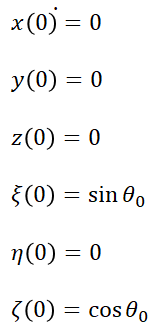and final conditions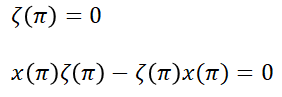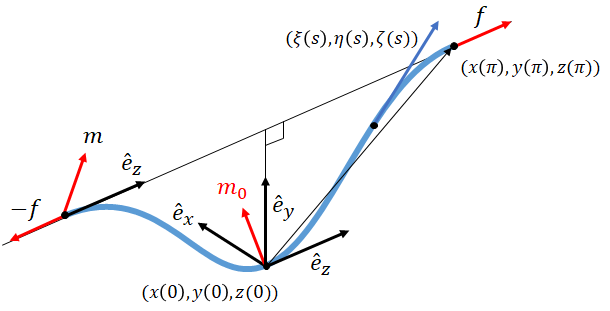That's six differential equations with six initial conditions and two constraints. There are four parameters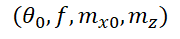so there are two degrees of freedom, and the solution manifold is a surface. We will plot it in the 3d projectionof the parameter space (because 4d is hard). Here is the surface looking down the f axis --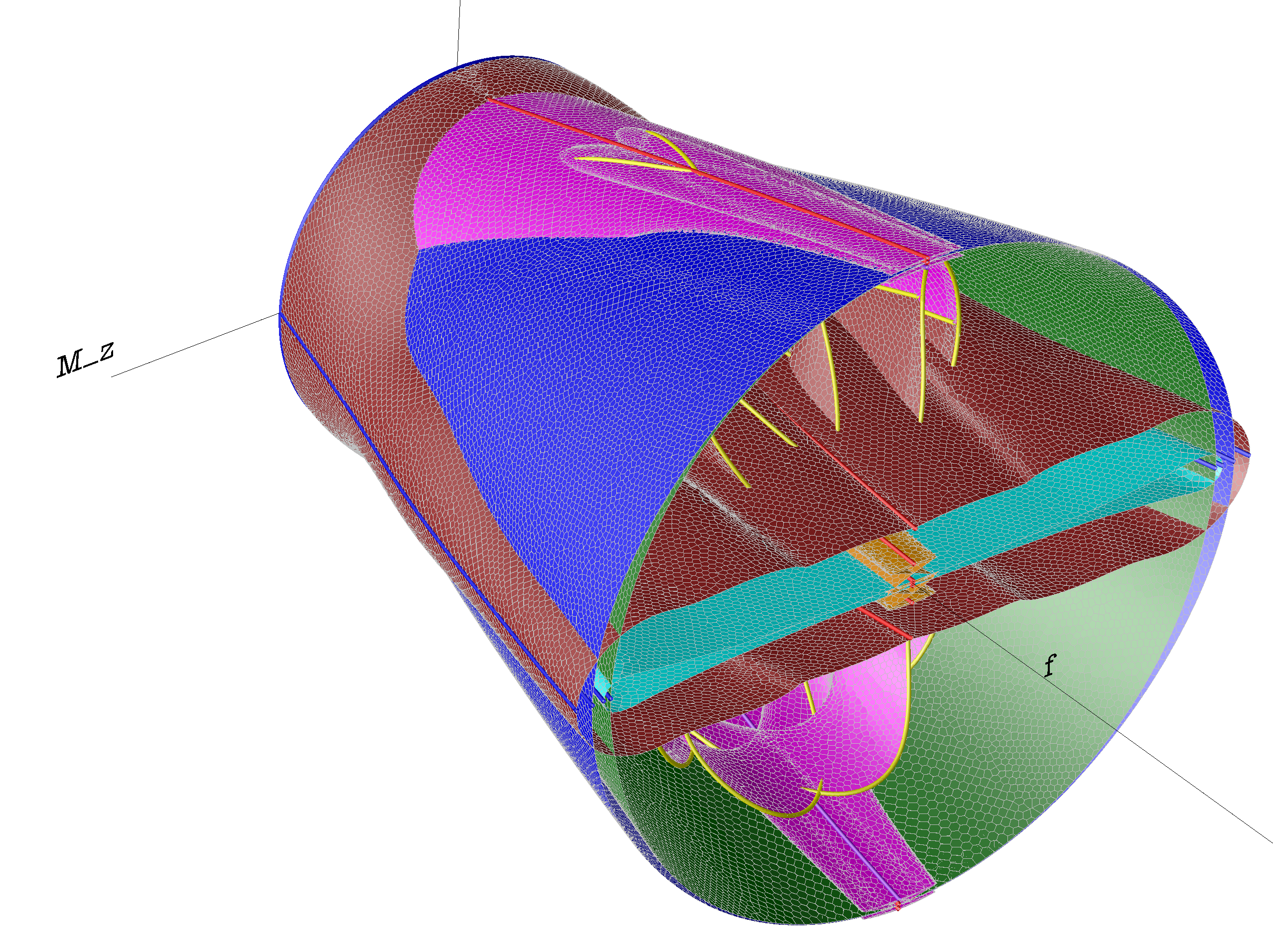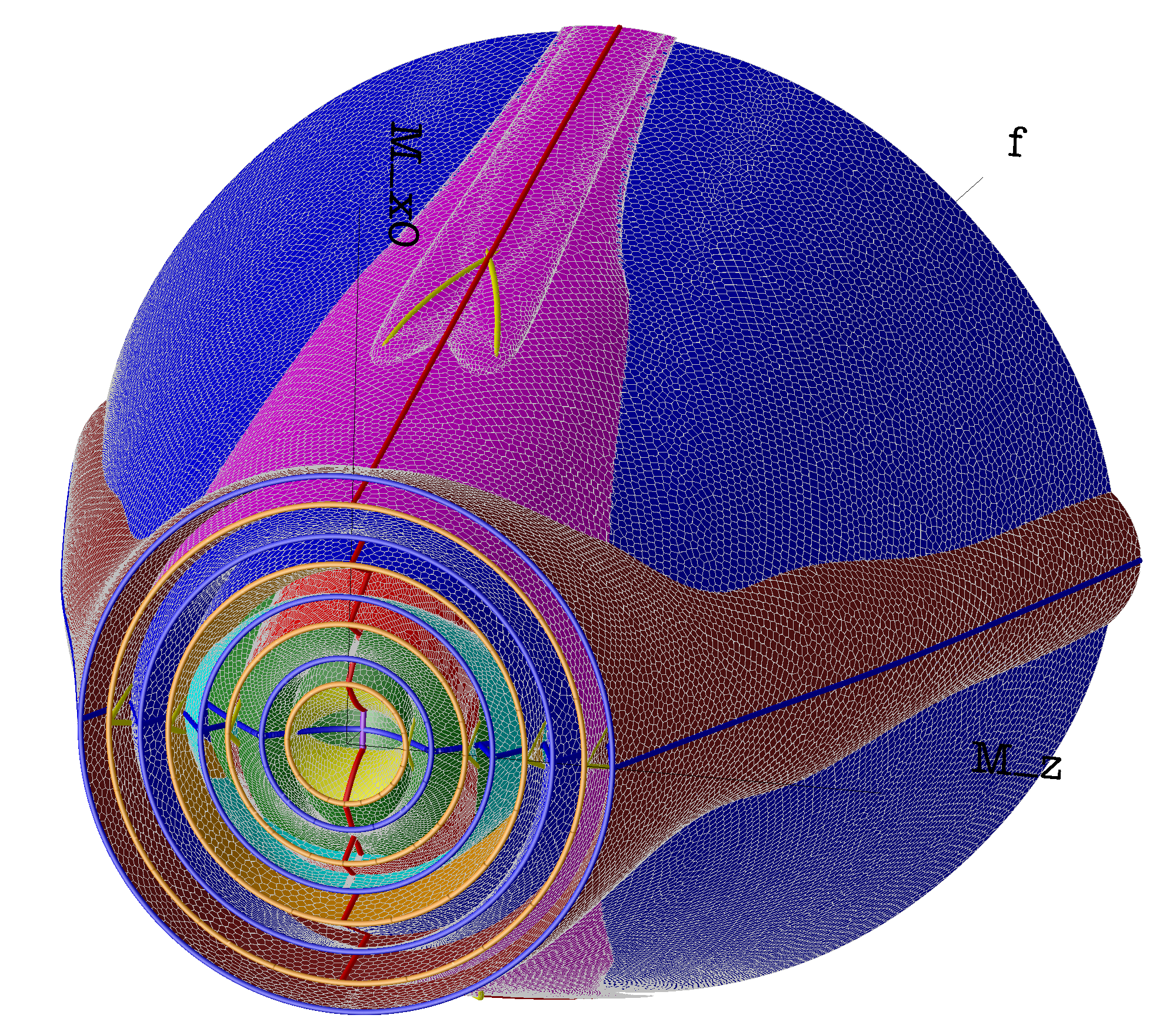The yellow lines are closed rings. This is one extra boundary condition, so the closed rings line on curves in the parameter space. Here the configurations near the closed ringsSebastien has a classification based on a winding number and an orientation, so that the surface is divided into regions like 4+ by the closed rings. Here are several of these subsurfaces (they go on infinitely in f)